## Tips

### Perio•• Model:P1
• Order Code:Z217404
••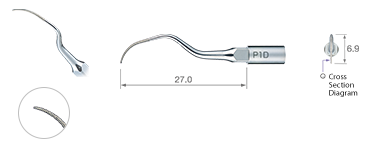• Model:P1D
• Order Code:Z217405

Diamond coated

•• Model:P2D
• Order Code:Z217402

Diamond coated / Right curved type

••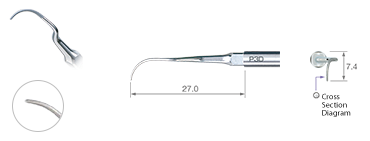• Model:P3D
• Order Code:Z217403

Diamond coated / Left curved type

••• Model:P10
• Order Code:Z217410

Sharp edge

•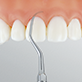•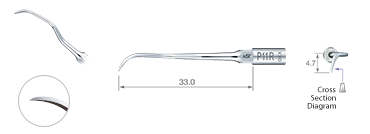• Model:P11R
• Order Code:Z217408

Sharp edge / Right curved type

•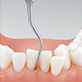•• Model:P11L
• Order Code:Z217409

Sharp edge / Left curved type

••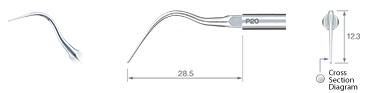• Model:P20
• Order Code:Z217420
• Model:P20-E
• Order Code:Z291420
•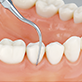•• Model:P21R
• Order Code:Z217422
• Model:P21R-E
• Order Code:Z291422

Right curved type

••• Model:P21L
• Order Code:Z217421
• Model:P21L-E
• Order Code:Z291421

Left curved type

•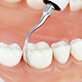•• Model:P25R
• Order Code:Z217424
• Model:P25R-E
• Order Code:Z291424

Right curved type

••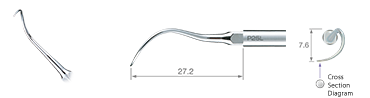• Model:P25L
• Order Code:Z217425
• Model:P25L-E
• Order Code:Z291425

Left curved type

•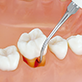•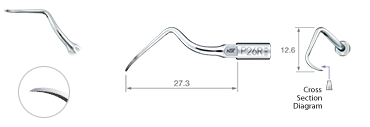• Model:P26R
• Order Code:Z217426

Right curved type

•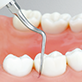•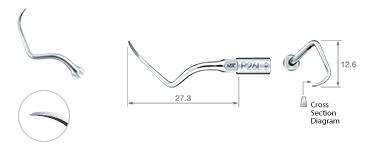• Model:P26L
• Order Code:Z217427

Left curved type

••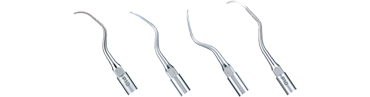• Model:Perio Kit A
• Order Code:Y900144

P1D, P2D, P3D, P10 : 1 pc. each / Tip holder : 1 pc.

•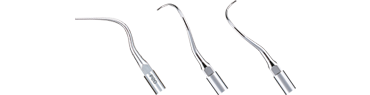• Model:Perio Kit B
• Order Code:Y900145

P20, P25R, P25L : 1 pc. each / Tip holder : 1 pc.